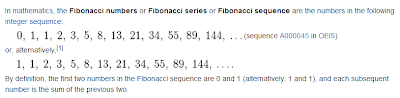# Fibonacci Sequence With and Without Recursion

Fibonacci sequence is sequence with subsequent number is the sum of the previous two. See snapshot below from wikipedia.We can implement this algorithm either using recursion or without. Let see both.Before the implementation let see how is the sequence defined for every F(n).

F(0) = 1 or F(0) = 0 in some definition
F(1) = 1
F(n) = F(n-1) + F(n-2)

Now these are implementation using Java

With Recursion

int fibo(int in){

if(n <= 1){

return n

}else{

return fibo(n-1) + fibo(n-2);

}
}


Without Recursion

int fibo(int n){

if(n <= 1){

return n;

}

int fibo = 1;

int fiboPrev = 1;

for(int i = 2; i < n; ++i){

int temp = fibo;

fibo += fiboPrev;

fiboPrev = temp;

}

return fibo;
}